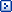Conversion tables A-KConversion tables L-ZSPAM database queryReverse WHOIS utilityNorth America area codesPHP Example forums

PHP example repository.

 Web www.web-max.ca
 Back to examples Basic multi-purpose conversion table script The following is a simple template to create a conversion table. It allow for the loading of multiple conversion equations into an array. Obviously, these equations could be loaded from a flat text file or a database. The equations are stored in an array and are parsed in the final calculation using the eval() function. This allows for complex functions to be stored. The equation in the array is the part before the equals sign. The part after the equals sign is a description of the conversion to take place. The value to be converted is added to the code by replacing the # in the array with the value. We could have stored the complete code in the array ( ie "\$value=\$value*0.3" ) but I prefer this method as it gives me the flexability to export the array to other languages ( mainly if I am using a text file to store the formulas ). You can see a working version of the conversion tables here. ``` ``` Example